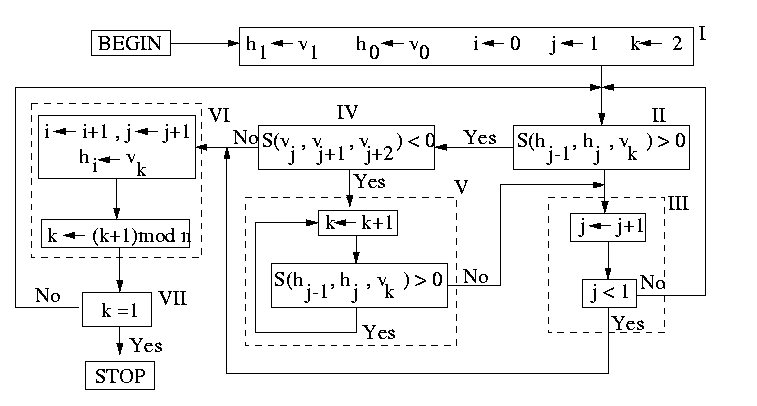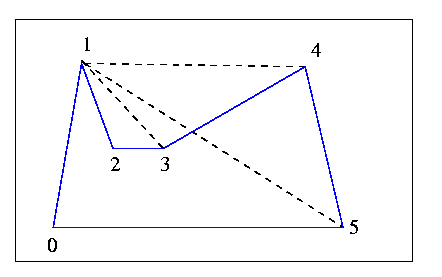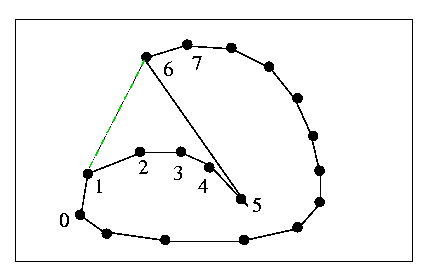Chen 1989

As mentioned in the introduction, Melkman's algorithm is generally considered to be the best one. It is simple, short, and on-line. Chen presented an algorithm  two years later, but seemed to be unaware of Melkman's work.

Chen describes the conditions that lead to the failure of Sklansky's original algorithm, and then proposes to handle these conditions. The author presents a box-diagram which describes the algorithm, and an example polygon with all the operations performed. The diagram of the algorithm is shown below. It is assumed that the polygon is ordered clockwise. Vertices are denoted by vi, with (i) ranging from 0 to n-1. The first vertex of index 0 must be an extreme vertex. The convex hull vertices are stored in a stack, which has h0 at the bottom and hJ at the top. The current vertex being examined is K, and the vertex which comes before hJ on the polygon is marked by i. The function S(x,y,z) is positive if (x,y,z) form a left turn.Soon after Chen published this algorithm, Toussaint provided a simple counterexample . He stated that in Chen's diagram "Box IV is rather mysterious and seems superfluous". The counterexample is shown below. You may easily verify that the algorithm in the diagram will skip through all vertices after 1. Toussaint also points out that there is no stopping criterion in this situation.Box IV diagram makes little sense to me. The variable j is intended to keep track of the top of the stack. It should not be used with the vertices, v. The algorithm is supposed to process the neighborhood of the top of the stack, which could potentially be nowhere near the vertices vJ,vJ+1,vJ+2 of Box IV. Imagine that the polygon begins with a long convex chain, so that the algorithm moves through boxes II, IV, VI repeatedly (see figure below). Then let there be one polygon edge (5,6) which causes the algorithm to backtrack almost to the beginning. Then let it continue with only convex turns to the end. At vertex 5, the variables i and j will have only been incremented. At vertex 6, where the backtracking of Box III occurs, j will be decremented down to 1. Then the algorithm should just move along the remaining convex section (from 1 to 6 to 7...). But box IV will still be checking vertices within the pocket, since j has been brought back down to 1. As soon as the second convex region is encountered, box IV will still be checking local convexity within the "pocket".Chen associates Box IV with his lemma 3.4 and 3.5, which deal with being inside pockets. Therefore, it seems that his diagram does not do what he intended it to do. It seems to me that if we replace the j's with i's in Box IV, and add one incrementing box for the variable (i) in Box III, we get the algorithm of Shin and Woo. This is quite a coincidence. As far as I know, Chen has not stepped forward to declare that there was a clerical error in the diagram after Toussaint's counterexample was published. Even if this were true, what is the point of repeating the algorithm of Shin and Woo? Chen even makes reference to it in the introduction.

previous : Melkman 1987.          next : Other algorithms.